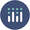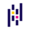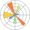# Scatterplot

A scatter plot displays the relationship between 2 numeric variables, one being displayed on the X axis (horizontal) and the other on the Y axis (vertical). Each data point is represented as a circle.

Several tools allow to build scatterplots in python. Seaborn is probably the most straightforward library for the job, but matplotlib allows a greater level of customization. If you are looking for an interactive chart, plotly is definitely the way to go.

This page provides many examples of scatterplots made with those Python tools, going from simple examples to highly customized versions.

## ⏱ Quick start (Seaborn)

``````# library & dataset
import seaborn as sns

# use the function regplot to make a scatterplot
sns.regplot(x=df["sepal_length"], y=df["sepal_width"])
``````

## ⏱ Quick start (Matplotlib)

``````# libraries
import matplotlib.pyplot as plt
import numpy as np
import pandas as pd

# Create a dataset:
df=pd.DataFrame({'x_values': range(1,101), 'y_values': np.random.randn(100)*15+range(1,101) })

# plot
plt.plot( 'x_values', 'y_values', data=df, linestyle='none', marker='o')
plt.show()
``````

##Scatterplots with `Plotly`

If you are looking for an interactive scatterplot, plotly is definitely the way to go.

Interactivity is a real plus for scatterplots. It is very useful to have a tooltip associated to every markers to get some additional information about it. Zooming on a specific area of the scatterplot is also very valuable sometimes.

The examples below should help you get started quickly with the plotly API:

##Scatterplots with `Pandas`

Pandas, a data analysis library, also offers functions to build scatterplots. It uses matplotlib under the hood, but the syntax is more concise.

The main difference is that we have to work with Pandas objects such as `Series` and `DataFrame`.

The examples below should help you get started quickly with the pandas API:

##Best python scatterplot examples

The web is full of astonishing charts made by awesome bloggers, (often using R). The Python graph gallery tries to display (or translate from R) some of the best creations and explain how their source code works.

The first example below demos how to add clean labels on a scatterplot, automatically avoiding overlapping. It also explains how to control background, fonts, titles and more.

If you want to display your work here, please drop me a word or even better, submit a Pull Request!

## Contact

👋 This document is a work by Yan Holtz. You can contribute on github, send me a feedback on twitter or subscribe to the newsletter to know when new examples are published! 🔥